# Neuroscience Lab: a tour through the eyes of a pythonista, PyData Barcelona2017

May. 23, 2017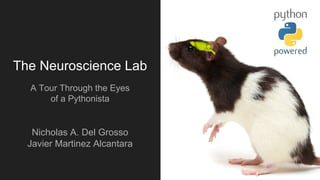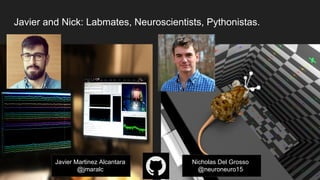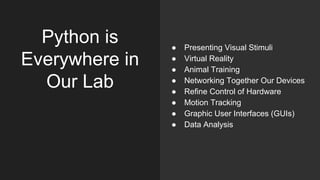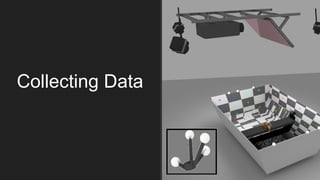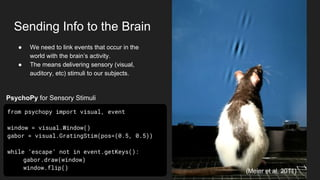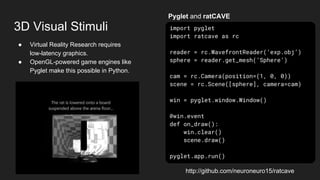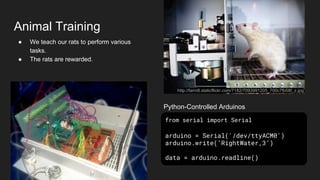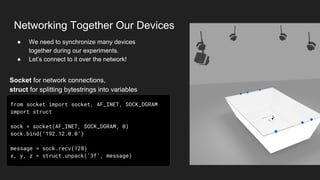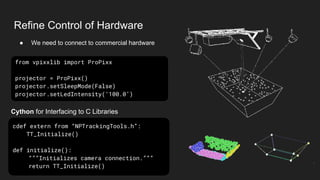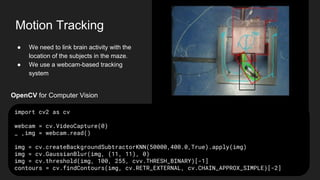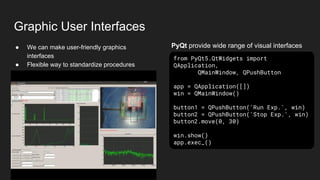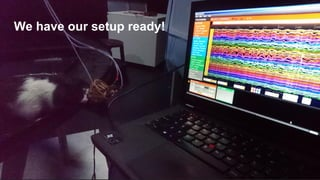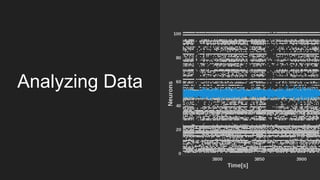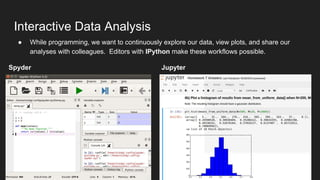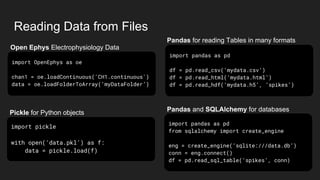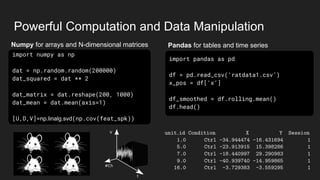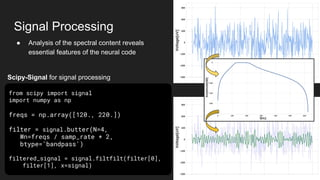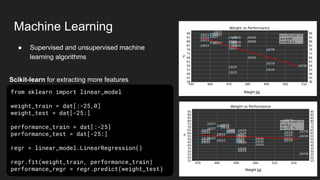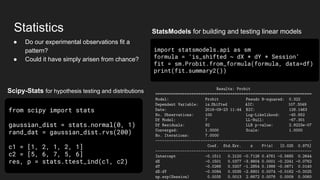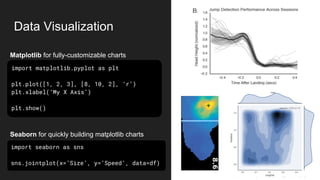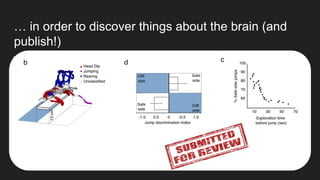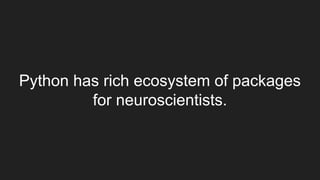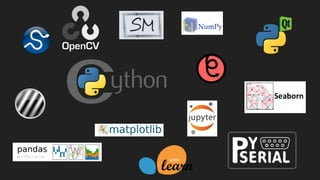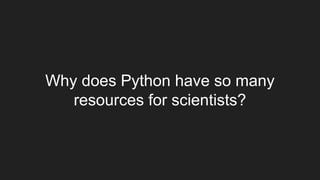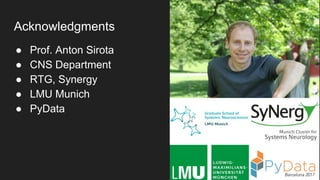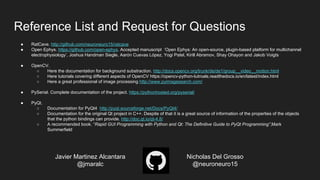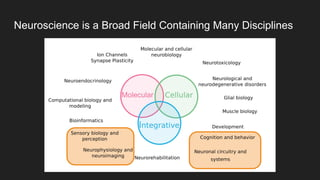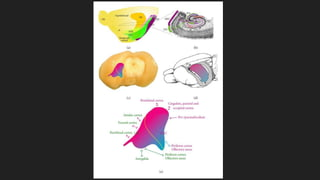1 of 30

### Neuroscience Lab: a tour through the eyes of a pythonista, PyData Barcelona2017

• 1. The Neuroscience Lab A Tour Through the Eyes of a Pythonista Nicholas A. Del Grosso Javier Martinez Alcantara
• 2. Javier and Nick: Labmates, Neuroscientists, Pythonistas. Javier Martinez Alcantara @jmaralc Nicholas Del Grosso @neuroneuro15
• 3. Python is Everywhere in Our Lab ● Presenting Visual Stimuli ● Virtual Reality ● Animal Training ● Networking Together Our Devices ● Refine Control of Hardware ● Motion Tracking ● Graphic User Interfaces (GUIs) ● Data Analysis
• 4. Collecting Data
• 5. Sending Info to the Brain ● We need to link events that occur in the world with the brain’s activity. ● The means delivering sensory (visual, auditory, etc) stimuli to our subjects. from psychopy import visual, event window = visual.Window() gabor = visual.GratingStim(pos=(0.5, 0.5)) while ‘escape’ not in event.getKeys(): gabor.draw(window) window.flip() (Meier et al, 2011) PsychoPy for Sensory Stimuli
• 6. 3D Visual Stimuli import pyglet import ratcave as rc reader = rc.WavefrontReader(‘exp.obj’) sphere = reader.get_mesh(‘Sphere’) cam = rc.Camera(position=(1, 0, 0)) scene = rc.Scene([sphere], camera=cam) win = pyglet.window.Window() @win.event def on_draw(): win.clear() scene.draw() pyglet.app.run() ● Virtual Reality Research requires low-latency graphics. ● OpenGL-powered game engines like Pyglet make this possible in Python. http://github.com/neuroneuro15/ratcave Pyglet and ratCAVE
• 7. Animal Training ● We teach our rats to perform various tasks. ● The rats are rewarded. from serial import Serial arduino = Serial('/dev/ttyACM0') arduino.write(‘RightWater,3’) data = arduino.readline() http://farm8.staticflickr.com/7182/7093991205_700c7fb58f_z.jpg Python-Controlled Arduinos
• 8. Networking Together Our Devices from socket import socket, AF_INET, SOCK_DGRAM import struct sock = socket(AF_INET, SOCK_DGRAM, 0) sock.bind(‘192.12.0.0’) message = sock.recv(128) x, y, z = struct.unpack(‘3f’, message) ● We need to synchronize many devices together during our experiments. ● Let’s connect to it over the network! Socket for network connections, struct for splitting bytestrings into variables
• 9. Refine Control of Hardware ● We need to connect to commercial hardware cdef extern from “NPTrackingTools.h”: TT_Initialize() def initialize(): “””Initializes camera connection.””” return TT_Initialize() Cython for Interfacing to C Libraries from vpixxlib import ProPixx projector = ProPixx() projector.setSleepMode(False) projector.setLedIntensity(‘100.0’)
• 10. Motion Tracking import cv2 as cv webcam = cv.VideoCapture(0) _ ,img = webcam.read() img = cv.createBackgroundSubtractorKNN(50000,400.0,True).apply(img) img = cv.GaussianBlur(img, (11, 11), 0) img = cv.threshold(img, 100, 255, cvv.THRESH_BINARY)[-1] contours = cv.findContours(img, cv.RETR_EXTERNAL, cv.CHAIN_APPROX_SIMPLE)[-2] ● We need to link brain activity with the location of the subjects in the maze. ● We use a webcam-based tracking system OpenCV for Computer Vision
• 11. Graphic User Interfaces ● We can make user-friendly graphics interfaces ● Flexible way to standardize procedures from PyQt5.QtWidgets import QApplication, QMainWindow, QPushButton app = QApplication([]) win = QMainWindow() button1 = QPushButton('Run Exp.', win) button2 = QPushButton('Stop Exp.', win) button2.move(0, 30) win.show() app.exec_() PyQt provide wide range of visual interfaces
• 12. We have our setup ready!
• 13. Analyzing Data
• 14. Interactive Data Analysis Spyder Jupyter ● While programming, we want to continuously explore our data, view plots, and share our analyses with colleagues. Editors with IPython make these workflows possible.
• 15. import OpenEphys as oe chan1 = oe.loadContinuous('CH1.continuous') data = oe.loadFolderToArray(‘myDataFolder’) import pandas as pd from sqlalchemy import create_engine eng = create_engine(‘sqlite:///data.db’) conn = eng.connect() df = pd.read_sql_table(‘spikes’, conn) Reading Data from Files import pandas as pd df = pd.read_csv(‘mydata.csv’) df = pd.read_html(‘mydata.html’) df = pd.read_hdf(‘mydata.h5’, ‘spikes’) import pickle with open(‘data.pkl’) as f: data = pickle.load(f) Pandas for reading Tables in many formats Pandas and SQLAlchemy for databasesPickle for Python objects Open Ephys Electrophysiology Data
• 16. Powerful Computation and Data Manipulation Pandas for tables and time seriesNumpy for arrays and N-dimensional matrices import numpy as np dat = np.random.random(200000) dat_squared = dat ** 2 dat_matrix = dat.reshape(200, 1000) dat_mean = dat.mean(axis=1) [U,D,V]=np.linalg.svd(np.cov(feat_spk)) import pandas as pd df = pd.read_csv(‘ratdata1.csv’) x_pos = df[‘x’] df_smoothed = df.rolling.mean() df.head()
• 17. Signal Processing from scipy import signal import numpy as np freqs = np.array([120., 220.]) filter = signal.butter(N=4, Wn=freqs / samp_rate * 2, btype='bandpass') filtered_signal = signal.filtfilt(filter, filter, x=signal) ● Analysis of the spectral content reveals essential features of the neural code Scipy-Signal for signal processing
• 18. Weight [g] Machine Learning ● Supervised and unsupervised machine learning algorithms from sklearn import linear_model weight_train = dat[:-25,0] weight_test = dat[-25:] performance_train = dat[:-25] performance_test = dat[-25:] regr = linear_model.LinearRegression() regr.fit(weight_train, performance_train) performance_regr = regr.predict(weight_test) Scikit-learn for extracting more features Weight [g]
• 19. Statistics Scipy-Stats for hypothesis testing and distributions StatsModels for building and testing linear models from scipy import stats gaussian_dist = stats.normal(0, 1) rand_dat = gaussian_dist.rvs(200) c1 = [1, 2, 1, 2, 1] c2 = [5, 6, 7, 5, 6] res, p = stats.ttest_ind(c1, c2) import statsmodels.api as sm formula = ‘is_shifted ~ dX * dY * Session’ fit = sm.Probit.from_formula(formula, data=df) print(fit.summary2()) ● Do our experimental observations fit a pattern? ● Could it have simply arisen from chance?
• 20. Data Visualization Matplotlib for fully-customizable charts Seaborn for quickly building matplotlib charts import matplotlib.pyplot as plt plt.plot([1, 2, 3], [8, 10, 2], ‘r’) plt.xlabel(‘My X Axis’) plt.show() import seaborn as sns sns.jointplot(x=’Size’, y=’Speed’, data=df)
• 21. … in order to discover things about the brain (and publish!)
• 22. Python has rich ecosystem of packages for neuroscientists.
• 24. Why does Python have so many resources for scientists?
• 25. It’s the community.
• 26. Acknowledgments ● Prof. Anton Sirota ● CNS Department ● RTG, Synergy ● LMU Munich ● PyData
• 27. Reference List and Request for Questions ● RatCave. http://github.com/neuroneuro15/ratcave ● Open Ephys. https://github.com/open-ephys. Accepted manuscript ‘Open Ephys: An open-source, plugin-based platform for multichannel electrophysiology’, Joshua Handman Siegle, Aarón Cuevas López, Yogi Patel, Kirill Abramov, Shay Ohayon and Jakob Voigts ● OpenCV. ○ Here the documentation for background substraction. http://docs.opencv.org/trunk/de/de1/group__video__motion.html ○ Here tutorials covering different aspects of OpenCV https://opencv-python-tutroals.readthedocs.io/en/latest/index.html ○ Here a great professional of image processing http://www.pyimagesearch.com/ ● PySerial. Complete documentation of the project. https://pythonhosted.org/pyserial/ ● PyQt. ○ Documentation for PyQt4 http://pyqt.sourceforge.net/Docs/PyQt4/ ○ Documentation for the original Qt project in C++. Despite of that it is a great source of information of the properties of the objects that the python bindings can provide. http://doc.qt.io/qt-4.8/ ○ A recommended book. “Rapid GUI Programming with Python and Qt: The Definitive Guide to PyQt Programming”,Mark Summerfield Javier Martinez Alcantara @jmaralc Nicholas Del Grosso @neuroneuro15
• 28. Spare Slides
• 29. Neuroscience is a Broad Field Containing Many Disciplines